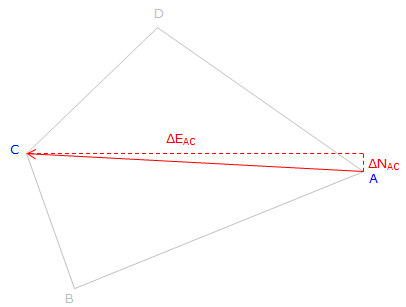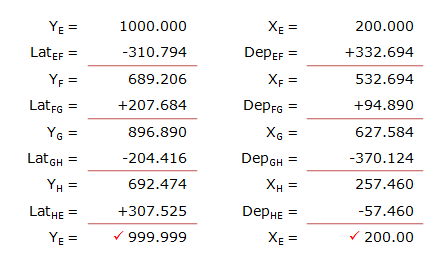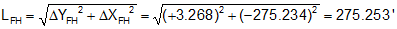## 3. Examples

### a. Traverse 1

#### (1) Forward ComputationFigure F-10 Loop Traverse Forward Computation

 Adjusted Line Lat (ft) Dep (ft) AB -176.386 -438.574 BC +203.382 -73.105 CD +192.340 +198.635 DE -219.336 +313.044

The coordinates of point A are 500.000' N, 2000.000' E. Compute the coordinates of the remaining points.

A simple way is to set up a table with North coordinates and latituded in one column, East coordinates and depatures in another.#### (2) Inverse Computation

What are the length ad bearing of the line A to C in the diagram below?Figure F-11 Length anf Direction Determination

From Equation (F-4)Substituting into Equation (F-5)and Equation (F-6)Because ΔN is North and ΔE is West: N86°58'47.6"W

Line AC: 512.39' at N86°58'48"W

### b. Traverse 2

#### (1) Forward Computation

The crossing traverse in Figure F-12 was previously adjusted with the results shown below.Figure F-12 Crossing Traverse Forward ComputationThe coordinates of point E are 200.000' X, 1000.000' Y.

Compute the coordinates of the remaining points.

Arranging the computations in a table:#### (2) Inverse Computation

Determine the length and azimuth of the line from point F to point H.

Remember: Y=>N, X=>E; and it's To minus From.Because ΔY is North and ΔX is West:Line FH: 275.25' and azimuth of 270°40'49".